# An Easy to Understand Explanation of the Burrows Wheeler Transform

Data Compression is one of the key components that has allowed an explosion in communications and the ability to store massive amounts of data.

There are many ways to compress data, however, one of the most elegant and effective is the Burrows-Wheeler Transform. In this article I will explain how the Burrows-Wheeler Transform works and offer an easy to understand java implementation.

## The Theory

An Overview of Burrows-Wheeler
The Burrows-Wheeler Transform is a text transformation that takes an input string and produces an output string with a high amount of repeating characters. For example the word “banana” is transformed into “annb\$aa” ( the “\$” will be explained shortly). This output can be efficiently compressed.

The magic of Burrows-Wheeler is it’s ability to create output that contains long runs of identical characters and it’s ability to transform the output back to it’s original state. I’ll explain how this works in two sections, Burrows-Wheeler Forward and Burrows-Wheeler Back.

## Burrows Wheeler Forward

How Burrows Wheeler Works:
Suppose that we want to transform a string, s, to it’s burrows wheeler forward output. In this example we’ll let s = “banana”. Here are the steps:

1. Insert a mark character at the beginning. Suppose mark=”\$”. String s would now be “\$banana”

2.  Create the cyclic shifts of s.

\$banana
a\$banan
na\$bana
ana\$ban
nana\$ba
anana\$b
banana\$

3. Sort the cyclic shifts

\$banana
a\$banan
ana\$ban
anana\$b
banana\$
na\$bana
nana\$ba

4. The Burrows-Wheeler output is the last column. output=“annb\$aa”

That’s it!  In java, it takes about 10 lines of code to write.  The repeats in the output string can be efficiently compressed with algorithms such as Zero Running, Move-to-front and Huffman Coding ( for more information on making a BW based compression algorithm that’s comparable in performance to gzip check out http://james.fabpedigree.com/bwt.htm )

Why Burrows Wheeler Works
How does BW create so many repeats? The idea is pretty simple. When the cyclic shifts are sorted they tend to produce runs of alphabetically similar suffices. Many of these suffices are predicted by the character that proceeds them (the characters located in the last column of the sorted shifts). For example in the word banana, the letter “a” is often followed by the letter “n”. The letter “n” is often followed by the letter “a”. This happens a lot in the english language. Heres another example.

Suppose you were compressing this string:

“\$the fox went to the hole and then to the apples”

In the sorted cyclic shifts their would be a long stretch of “t”s. The following is portion from the sorted cyclic shifts:

he apples \$the fox went to the hole and then to t
he fox went to the hole and then to the apples \$t
he hole and then to the apples \$the fox went to t
hen to the apples \$the fox went to the hole and t

The repeating “t”s occur because the suffix “he” is usually proceeded by a “t”. Of course this doesn’t always work. Consider this string:

“\$the she fox went to the hole and then to the apples”

he apples \$the she fox went to the hole and then to t
he fox went to the hole and then to the apples \$the s
he hole and then to the apples \$the she fox went to t
he she fox went to the hole and then to the apples \$t
hen to the apples \$the she fox went to the hole and t
The long run of “t”s is now interrupted by an “s”. The interruption makes it harder to compress however their is still a run of 3 “t”s.

## Burrows Wheeler Back (Inverse)

The forward BW transform creates output that has an incredible amount of repeating characters. The big question is can we recover the original string? The answer is yes. Furthermore we can do in it in linear time! In this section I’ll explain how and why the BW inverse works.

Lets say we have BW forward output, string s, that we want to transform back to the original string. Say s equals “annb\$aa”, the output of the previous section. With this we can produce the first(F) and last(L) columns of our sorted cyclic shifts in the forward transform. To do this we must sort s.

F                L
\$   banan   a
a   \$bana   n
a   na\$ba   n
a   nana\$   b
b   anana   \$
n   a\$ban   a
n   ana\$b   a

Note: The greyed-out center part is unknown, but shown for clarity.

Now we have all the pairs of letters in our original word:

Pairs
\$a
an
an
ab
b\$
na
na

(The pairs are in reverse order but we can reverse our output at the last step before returning the string.)

So what can we do now? It looks like you could “chain” the word banana back together from this output. Start with the “\$” character and work backwards.Reverse the output and viola. “banana” has been recovered! Unfortunately this method doesn’t always work. You need to know the correct way to put the pairs back together. Suppose we wanted to transform the word “banaxna” with Burrows-Wheeler. The forward transform goes like this:
If we tried to chain this together we would get something like this:
output = “baxna”

Although we know the pairs, we need to know how to put them back together in the correct order. Lets examine what went wrong to find a solution.

The problem we ran into when chaining “anbn\$xaa” together was the we selected the wrong pair that begin with an “a”. The below diagram shows our mistake.

From the sorted pairs:
an
ab < —  Choice we naively made.
an < — Choice we needed to make.

Luckily, we can figure out how to match each pair with the pair that follows it from just the first and last columns. We can figure out how to chain the pairs that end with “a”s to the pairs that begin with “a”s by noting that that the first “a” in L corresponds to the first “a” in F. The second “a” in L corresponds to the second “a” in F and so on. The pairs  are linked by order of appearance. The diagram below makes this obvious.
Why this works is not immediately obvious. The “a”s match up in a stable fashion because the remaining bodies of words that begin with an “a”( \$banaxn, naxna\$b, xna\$ban) are sorted in the same manner as the words that end in “a”s (\$banaxna, naxna\$ba, xna\$bana). They are sorted in the same order whether they are prefixed with an “a” or not. Again a diagram is useful to help explain: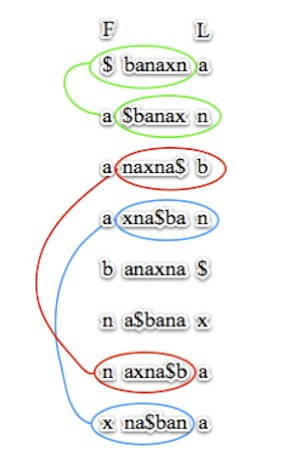Notice how “naxna\$b” comes before “xna\$ban” whether it is prefixed with an “a” or not.

With this knowledge we can chain “anbn\$xaa” correctly.

Note: OA = Order of Appearance (based on appearance in F and L)
Reverse it and the original word, “banaxna”, is recovered!

## The Code

Now that you see how it works, we’re ready for some code. The code can be broken down into three steps.

1. Create F and L
2. Find the “correct” way to chain the pairs back together
3. Chain the pairs together, reverse the output and return it.

Parameters of the “back” method: String bw, char mark.

Where bw is a string transformed by the forward Burrows-Wheeler method and mark is the marked character.
1. Create F and L
The easiest step! Not much to explain here.
``` char [] L = bw.toCharArray(); char [] F = bw.toCharArray(); Arrays.sort(F);```

2. Find the “Correct” Way to Chain the Pairs back Together
Although this is probably the hardest step, it’s not too bad. To create a path through we will use an int array, T, to match the last letter of a pair the corresponding first letter of the next pair. In other words L[i] = F[T[i]]

Here’s an illustration:
So the big question now is how to compute T[i]? I propose a two step process:

Discover Step: Iterate once through L. Learn the positions of every character and their order of appearance.

Match Step: Iterate once through F.  Match each character in F to it’s corresponding letter in L by order of appearance. Record this matching in T[i].

Discover Step Details:
For this step I suggest using a hash table of queues. Every time a character, c, is iterated over, look it up in the hashtable where the key equals c. If the return is null then create a new queue, and store it in the hash table where the key is c. Enqueue the value of i into the queue. This will not only store the location of the c but a queue inherently keeps track of the order of appearance.

The following diagram shows the hash table result after this loop.The corresponding code:
``` HashMap<Character,Queue> map = new HashMap<Character,Queue>(); for ( int i=0; i < L.length; i++){ char c =  L[i]; Queue<Integer> q = map.get(c); if (q == null){ q = new LinkedList<Integer>(); map.put(c,q); } q.add(i); } ```
Match Step Details:
This step is pretty simple. For each character in F, get the corresponding queue via the hashtable. T[i] equals the removed integer.
``` int[] T = new int[bw.length]; for (int i=0; i < F.length; i++){ char c = F[i]; Queue<Integer> q = map.get(c); Integer x = q.remove(); T[x] = i; } ```
3) Rebuild the Original Text
Now that we have T made we just need to build the string. We start at the mark character in F, follow the path given by T[i] and append new characters to our growing string until we reach the mark character again.

We use a StringBuilder to append the string (Concatenation with a String would require copying the contents every time the string is appended! )

The code:
``` int i = Arrays.binarySearch(F, mark); //building index i = T[i]; //skip the mark character StringBuilder sb = new StringBuilder(); while(true){ char c = F[i]; if (c == mark) break; sb.append(c); i = T[i]; } sb.reverse(); return sb.toString(); ```
And there you have it,  the Burrows-Wheeler Transform Forward and Back. I put the entire code below so you can play around with it. There are also text files to that you can play around with as well as a small script to check the that your code is working correctly.

Code:
BWT.java  (see code for details on how to use)

Sample Texts:
banana.txt (6 bytes)
banaxna.txt (7 bytes)
ghost.txt (6 kb)
mobydick.txt (1.2 mb)
pipi.txt (1 mb) — takes about a minute to run on 2.2ghz cpu due to the relatively slow, n*log(n),  Arrays.sort() method. Results may vary.

Shell Script to Check BWT Transform:
runbwt